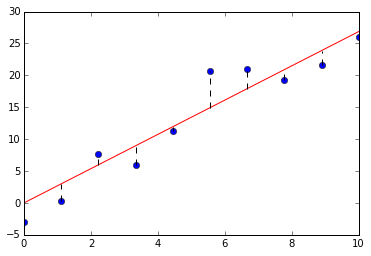# 优化

## 高阶导数（Higher Derivatives）from sympy.abc import x
from sympy.abc import y
f = x**2*y-2*x*y
# 求关于x的二次导数
print f.diff(x,2)
# result : 2*y
# 等同于反复关于x求两次导数
print f.diff(x).diff(x)
# result : 2*y
# 先求关于x的导数，再求关于y的导数，这个该怎么解读？
print f.diff(x,y)
# result : 2*(x - 1)


## 优化问题（Optimization Problem）

    plt.figure(1, figsize=(4,4))
plt.axis('off')
plt.axhspan(0,1,0.2,0.8,ec = "none")
plt.axhspan(0.2,0.8,0,0.2,ec = "none")
plt.axhspan(0.2,0.8,0.8,1,ec = "none")

plt.axhline(0.2,0.2,0.8,linewidth = 2, color = 'black')
plt.axvline(0.2,0.17,0.23,linewidth = 2, color = 'black')
plt.axvline(0.8,0.17,0.23,linewidth = 2, color = 'black')

plt.axvline(0.2,0.8,1,linewidth = 2, color = 'black')
plt.axhline(0.8,0.17,0.23,linewidth = 2, color = 'black')
plt.axhline(1,0.17,0.23,linewidth = 2, color = 'black')

plt.text(0.495,0.22,r"$l$", fontsize = 18,color="black")
plt.text(0.1,0.9,r"$\frac{4-l}{2}$", fontsize = 18,color="black")

plt.show()l = np.linspace(0,4,100)
V = lambda l: 0.5*l**2*(4-l)
plt.plot(l,V(l))## 关键点（Critical Points）

1. 如果，则函数处的函数值是局部最小值
2. 如果，则函数处的函数值是局部最大值
3. 如果，则测试无法告诉我们结论from sympy.abc import l
V = 0.5*l**2*(4-l)
# 看看一次导函数：
print V.diff(l)
# output is : -0.5*l**2 + 1.0*l*(-l + 4)
# 一次导函数的定义域为(-oo,oo),因此关键点为V'(l)=0的根
cp = sympy.solve(V.diff(l),l)
print cp
# output is: [0.0, 2.66666666666667]
# 找到关键点后，使用二次导数测试：
for p in cp:
print V.diff(l,2).subs(l,p)
# output is: 4, -4
# 因此知道在l=2.666666处时，纸盒的体积最大


## 线性回归（Linear Regression）

    # 设定好随机函数种子，确保模拟数据的可重现性
np.random.seed(123)

# 随机生成一些带误差的数据
x = np.linspace(0,10,10)
res = np.random.randint(-5,5,10)
y = 3*x + res

# 求解回归线的系数
a = sum(x*y)/sum(x**2)

# 绘图
plt.plot(x,y,'o')
plt.plot(x,a*x,'red')
for i in range(len(x)):
plt.axvline(x[i],min((a*x[i]+5)/35.0,(y[i]+5)/35.0),\
max((a*x[i]+5)/35.0,(y[i]+5)/35.0),linestyle = '--',\
color = 'black')slope, intercept = np.polyfit(x,y,1)
plt.plot(x,y,'o')
plt.plot(x,a*x,'red', linestyle = '--')
plt.plot(x,slope*x+intercept, 'blue')